# Graphing Quadratic Functions y 2 ax bx c

• Slides: 19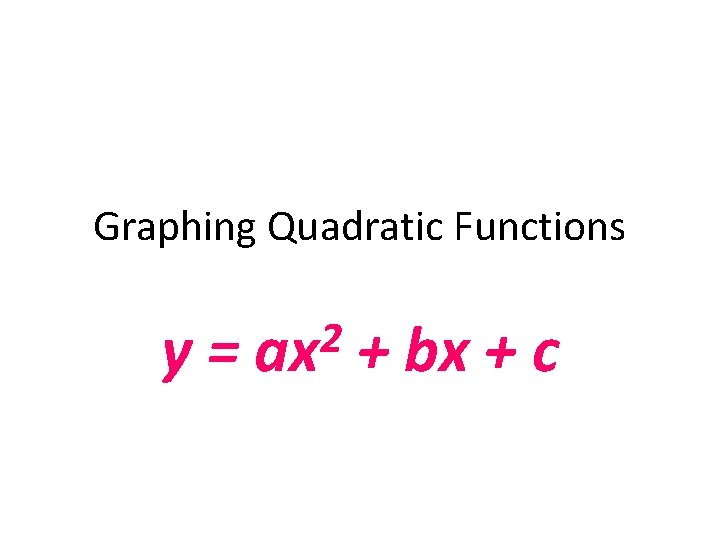Graphing Quadratic Functions y= 2 ax + bx + cGraphing Quadratic Functions Today we will: Understand how the coefficients of a quadratic function influence its graph a. b. c. d. e. The direction it opens (up or down) Its vertex Its line of symmetry Its y-intercepts Its x-intercepts (roots)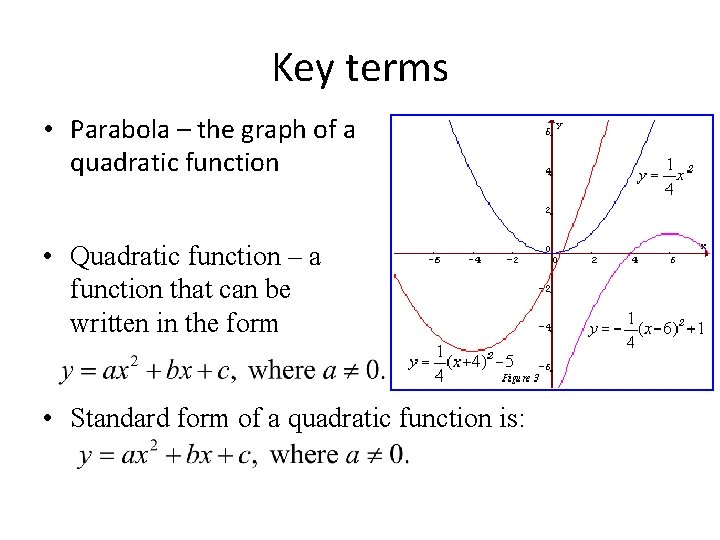Key terms • Parabola – the graph of a quadratic function • Quadratic function – a function that can be written in the form • Standard form of a quadratic function is: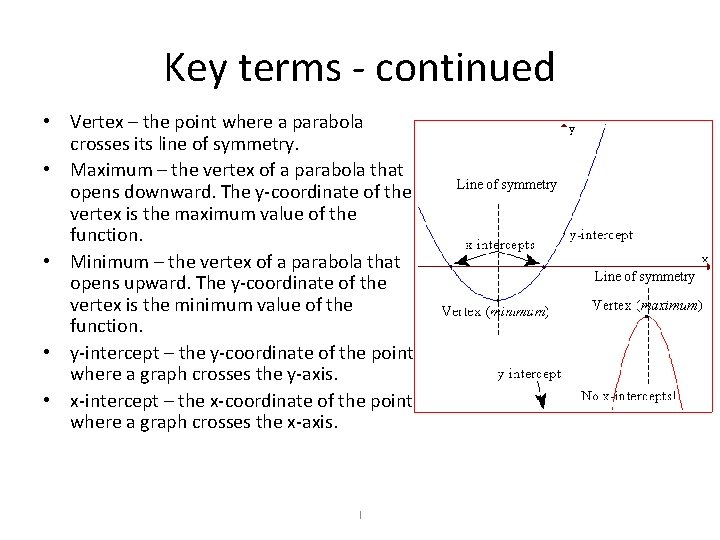Key terms - continued • Vertex – the point where a parabola crosses its line of symmetry. • Maximum – the vertex of a parabola that opens downward. The y-coordinate of the vertex is the maximum value of the function. • Minimum – the vertex of a parabola that opens upward. The y-coordinate of the vertex is the minimum value of the function. • y-intercept – the y-coordinate of the point where a graph crosses the y-axis. • x-intercept – the x-coordinate of the point where a graph crosses the x-axis. I Line of symmetryParabola y The graph of a quadratic function is a parabola. Vertex A parabola can open up or down. If the parabola opens up, the lowest point is called the vertex. x If the parabola opens down, the vertex is the highest point. Vertex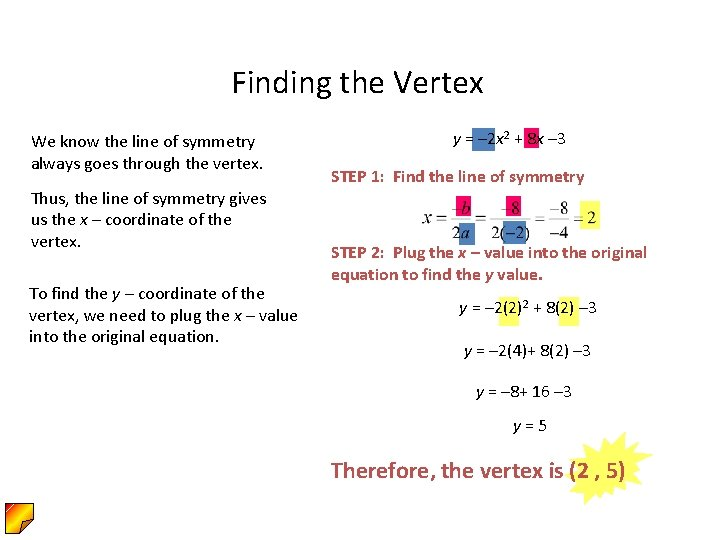Finding the Vertex We know the line of symmetry always goes through the vertex. Thus, the line of symmetry gives us the x – coordinate of the vertex. To find the y – coordinate of the vertex, we need to plug the x – value into the original equation. y = – 2 x 2 + 8 x – 3 STEP 1: Find the line of symmetry STEP 2: Plug the x – value into the original equation to find the y value. y = – 2(2)2 + 8(2) – 3 y = – 2(4)+ 8(2) – 3 y = – 8+ 16 – 3 y=5 Therefore, the vertex is (2 , 5)Vertex : Max & Min y The standard form of a quadratic function is a>0 y = ax 2 + bx + c The parabola will open up when the a value is positive. x The parabola will open down when the a value is negative. a<0Minimum and Maximum: Quadratic Functions • Consider f(x) = ax 2 + bx +c. 1. If a > 0, then f has a minimum that occurs at x = -b/(2 a). This minimum value is f(-b/(2 a)). 2. If a < 0, the f has a maximum that occurs at x = -b/(2 a). This maximum value is f(-b/(2 a)).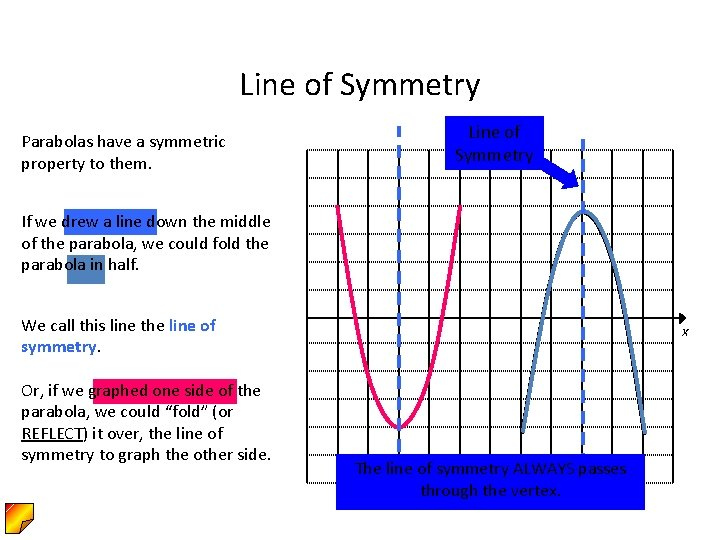Line of Symmetry Parabolas have a symmetric property to them. Line yof Symmetry If we drew a line down the middle of the parabola, we could fold the parabola in half. We call this line the line of symmetry. Or, if we graphed one side of the parabola, we could “fold” (or REFLECT) it over, the line of symmetry to graph the other side. x The line of symmetry ALWAYS passes through the vertex.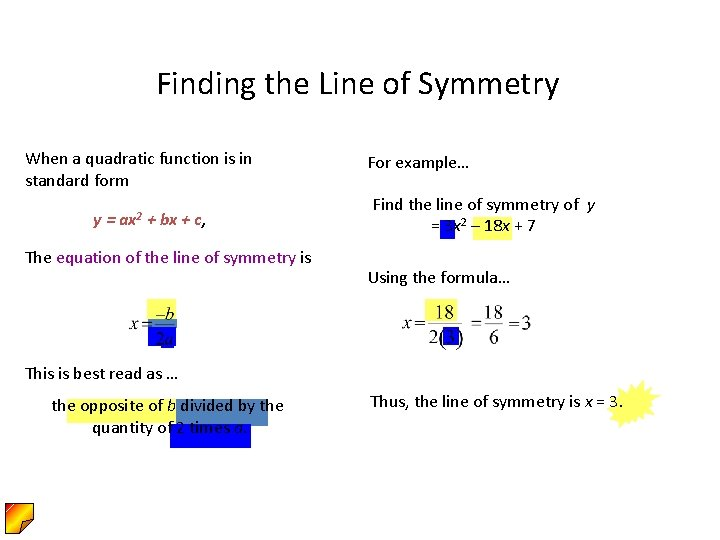Finding the Line of Symmetry When a quadratic function is in standard form y= ax 2 + bx + c, The equation of the line of symmetry is For example… Find the line of symmetry of y = 3 x 2 – 18 x + 7 Using the formula… This is best read as … the opposite of b divided by the quantity of 2 times a. Thus, the line of symmetry is x = 3.Graphing Parabolas With Equations in Standard Form To graph f (x) = y = ax 2 + bx + c: 1. Determine whether the parabola opens upward or downward. If a > 0, it opens upward. If a < 0, it opens downward. 2. Determine the vertex of the parabola. 3. Find any x-intercepts by replacing f (x) with 0. Solve the resulting quadratic equation for x. (Solving the functions when y = 0 ) 4. Find the y-intercept by replacing x with zero. 5. Plot the intercepts and vertex. Connect these points with a smooth curve that is shaped like a cup.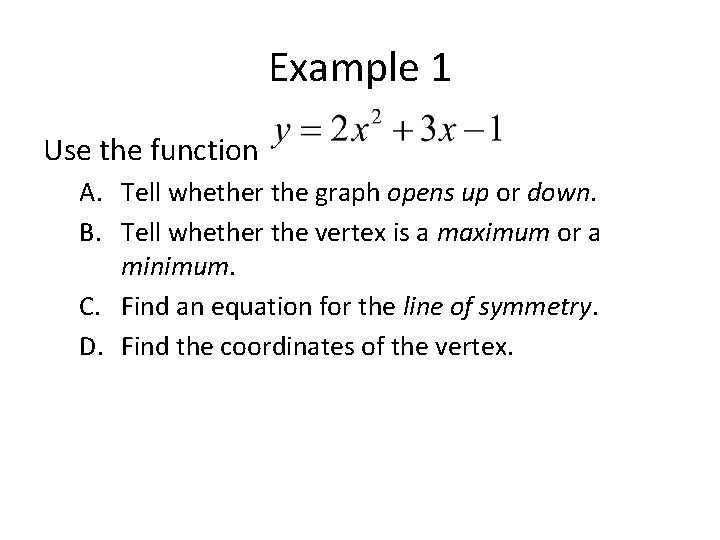Example 1 Use the function A. Tell whether the graph opens up or down. B. Tell whether the vertex is a maximum or a minimum. C. Find an equation for the line of symmetry. D. Find the coordinates of the vertex.Example 1 Solution Use the function A. a is positive, so the graph opens up. B. The vertex is a minimum. C. Equation for the line of symmetry. D. Coordinates of the vertex.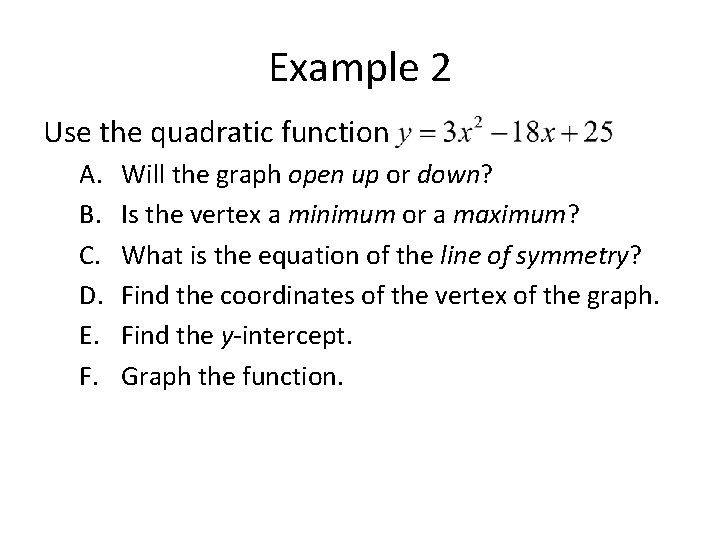Example 2 Use the quadratic function A. B. C. D. E. F. Will the graph open up or down? Is the vertex a minimum or a maximum? What is the equation of the line of symmetry? Find the coordinates of the vertex of the graph. Find the y-intercept. Graph the function.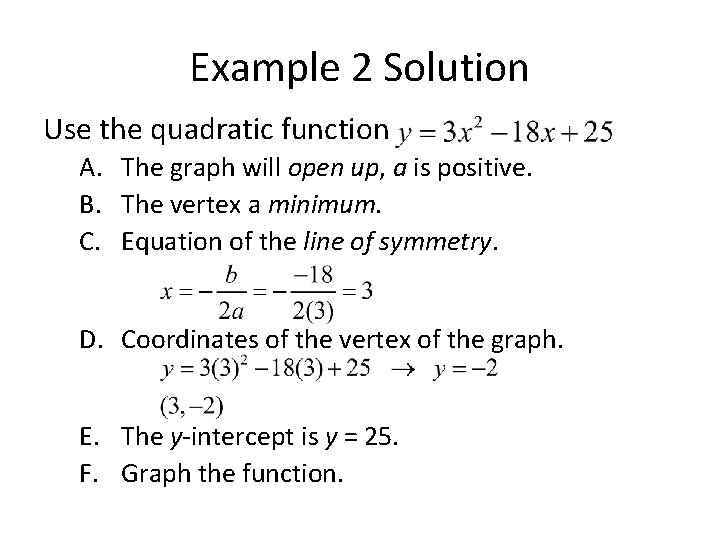Example 2 Solution Use the quadratic function A. The graph will open up, a is positive. B. The vertex a minimum. C. Equation of the line of symmetry. D. Coordinates of the vertex of the graph. E. The y-intercept is y = 25. F. Graph the function.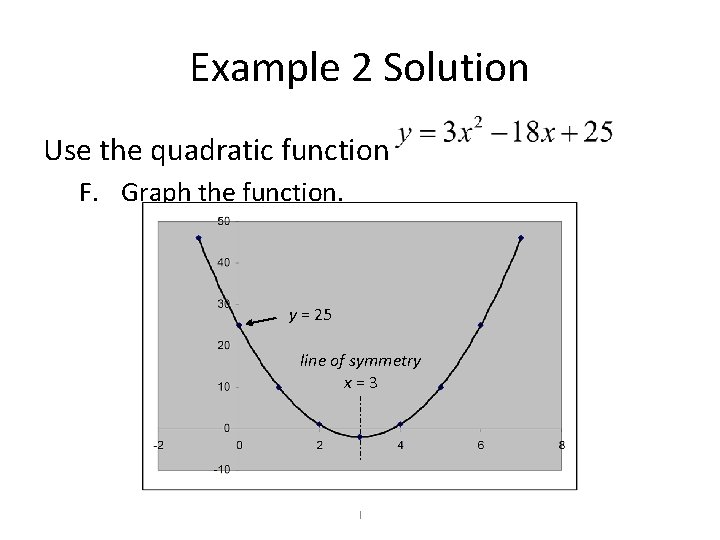Example 2 Solution Use the quadratic function F. Graph the function. y = 25 line of symmetry x=3 I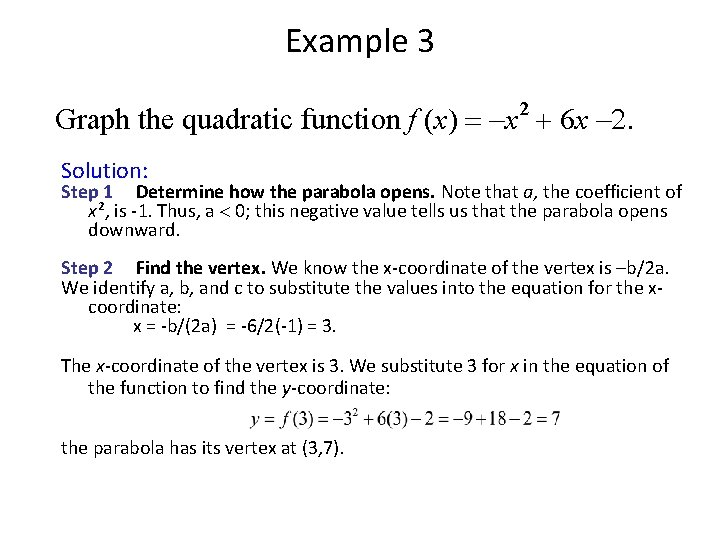Example 3 2 Graph the quadratic function f (x) = -x + 6 x -. Solution: Step 1 Determine how the parabola opens. Note that a, the coefficient of x 2, is -1. Thus, a < 0; this negative value tells us that the parabola opens downward. Step 2 Find the vertex. We know the x-coordinate of the vertex is –b/2 a. We identify a, b, and c to substitute the values into the equation for the xcoordinate: x = -b/(2 a) = -6/2(-1) = 3. The x-coordinate of the vertex is 3. We substitute 3 for x in the equation of the function to find the y-coordinate: the parabola has its vertex at (3, 7).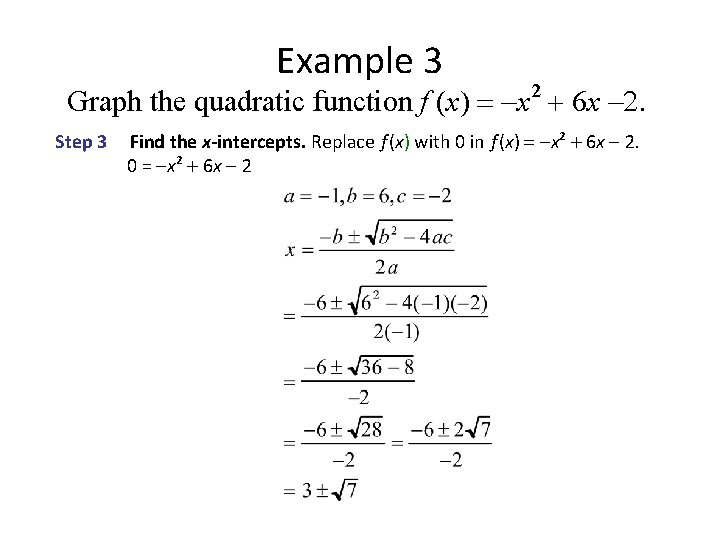Example 3 Graph the quadratic function f (x) = -x 2 + 6 x -. Step 3 Find the x-intercepts. Replace f (x) with 0 in f (x) = -x 2 + 6 x - 2. 0 = -x 2 + 6 x - 2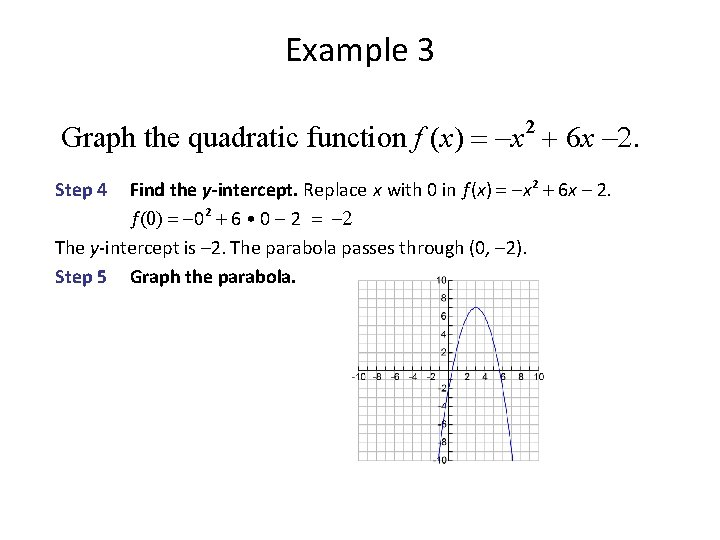Example 3 Graph the quadratic function f (x) = -x 2 + 6 x -. Find the y-intercept. Replace x with 0 in f (x) = -x 2 + 6 x - 2. f (0) = -02 + 6 • 0 - 2 = - The y-intercept is – 2. The parabola passes through (0, -2). Step 5 Graph the parabola. Step 4